• 运用Python的数组和矩阵操作模拟验证一阶自回归模型中，自回归系数OLS估计量的有限样本偏差问题。
• 使用场景多，多数情况下，就是调包，进行函数和数据的套入，然后得出各变量之间的参数。最后预测出一个结果。。 标准误差 标准误差是回归直线，即估计值和实际因变量值的平均平方误差。表示是SE。 可决系数 可决...
回归分析
回归分析的概念这里不多说了，她是一个很常见的机器学习算法。使用场景多，多数情况下，就是调包，进行函数和数据的套入，然后得出各变量之间的参数。最后预测出一个结果。。
标准误差
标准误差是回归直线，即估计值和实际因变量值的平均平方误差。表示是SE。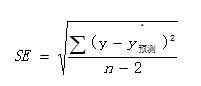可决系数
可决系数是衡量因变量和自变量直接关系密切程度的指标，表示自变量解释因变量变动的百分比。它取值范围是0到1之间。也叫拟合优度检验，系数也称为判断系数。公式为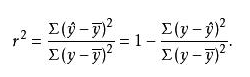相关系数
相关系数是用来测定变量之间的拟合优度的指标，计算公式为：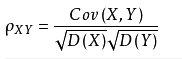即协方差和X与Y的方差平方根之积的比值。
回归系数显著性检验
对回归系数求出后，对回归系数进行显著性检验，回归系数的显著性检验用t参数进行检验。公式如下所示：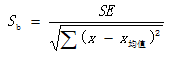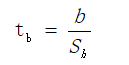F检验
将求解得到的Y和实际的Y值进行求差，得到总的离差，离差可分解为回归偏差和剩余残差两部分。于是将回归偏差和剩余残差各自除以它们的自由度后加以比较，便可得到检验统计量F。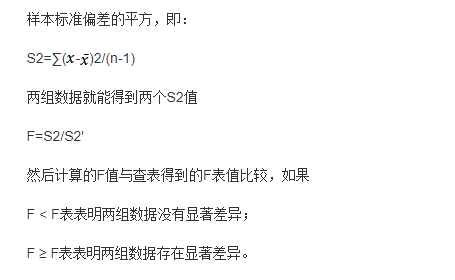德宾——沃森统计量
如果存在自相关问题，那么，用回归模型进行预测就要失真，德宾沃森统计量是检验模型是否存在自相关的一种有效方法。
展开全文• 逻辑回归模型已成为研究二进制响应变量之间的关联的常用方法。 它的广泛应用取决于其易于应用和... 这项工作将专注于评估拟合优度检验的渐近分布行为，还将进行全局拟合优度检验之间的比较，并通过仿真进行评估。
• 当影响因变量的因素是多个时候，这种一个变量同时与多个变量的回归问题就是多元回归，分为：多元线性回归和多元非线性回归。这里直说多元线性回归。对比一元线性回归： 1.1多元回归模型： y=β0+β1x1+β2x2+…+β...
当影响因变量的因素是多个时候，这种一个变量同时与多个变量的回归问题就是多元回归，分为：多元线性回归和多元非线性回归。这里直说多元线性回归。对比一元线性回归：
1.1多元回归模型：

y=β0+β1x1+β2x2+…+βkxk+ε

y=\beta_{0}+\beta_{1}x_{1}+\beta_{2}x_{2}+\ldots+\beta_{k}x_{k}+\varepsilon

1.2多元回归方程

E(y)=β0+β1x1+β2x2+…+βkxk

E(y)=\beta_{0}+\beta_{1}x_{1}+\beta_{2}x_{2}+\ldots+\beta_{k}x_{k}

1.3估计的多元回归方程

y^=β0^+β1^x1+β2^x2+…+βk^xk

\hat{y}=\hat{\beta_{0}}+\hat{\beta_{1}}x_{1}+\hat{\beta_{2}}x_{2}+\ldots+\hat{\beta_{k}}x_{k}
2.1**对参数的最小二乘法估计：**
和一元线性回归中提到的最小二乘法估计一样、这不过这里的求导变量多了点、原理是一样的、这里需要借助计算机求导、就不写了。

3 回归方程的拟合优度：
3.1 多重判定系数：(Multiple coefficient of determination)

R^{2}=\frac{SSR}{SST}=1-\frac{SSE}{SST}

注解：

(1 ) 对于多重判定系数有一点特别重要的需要说明：自变量个数的增加将影响到因变量中被估计的回归方程所解释的变量数量。当增加自变量时，会使预测误差变得较小，从而减小残差平方和

SSE
$SSE$。自然就会是

SSR
$SSR$变大。自然就会是

R2
$R^{2}$变大。这就会引发一个问题。如果模型中增加一个自变量，即使这个自变量在统计上并不显著，

R2
$R^{2}$的值也会变大。因此为了避免这个问题。提出了

R2a=1−(1−R2)(n−1n−k−1)

R^{2}_{a}=1-(1-R^{2})(\frac{n-1}{n-k-1})

R2a
$R^{2}_{a}$ 同时考虑了样本量

(n)
$(n)$ 和模型中自变量的个数

(k)
$(k)$ 的影响，这就使得

R2a
$R^{2}_{a}$ 的值永远小于

R2
$R^{2}$，而且

R2a
$R^{2}_{a}$ 的值不会因为模型中自变量的个数增多而逐渐接近于

1
$1$.
(2 ) R2$R^{2}$ 的平方根成为多重相关系数，也称为复相关系数，
它度量了因变量同

k
$k$ 个自变量的相关程度。
3.2 估计标准误差
同一元线性回归一样，多元回归中的估计标准误差也是误差项 ε$\varepsilon$ 的方差

σ2
$\sigma^{2}$ 的一个估计值，

se=SSEn−k−1−−−−−−−−√=MSE−−−−−√

s_{e}=\sqrt{\frac{SSE}{n-k-1}}=\sqrt{MSE} 4. 显著性检验 在此重点说明，在一元线性回归中，线性关系的检验

(F检验)
$(F检验)$ 和回归系数的检验

(t检验)
$(t检验)$ 是等价的。 但是在多元回归中，线性关系的检验主要是检验因变量同多个自变量线性关系是否显著，在

k
$k$ 个自变量中，只要有一个自变量与因变量的线性关系显著， F检验$F检验$ 就能通过，但这不一定意味着每个自变量与因变量的关系都显著。回归系数检验则是对每个回归系数分别进行单独的检验，它主要用于检验每个自变量对因变量的影响是否都显著。如果某个自变量没有通过检验，就意味着这个自变量对因变量的影响不显著，也许就没有必要将这个自变量放进回归模型中。  4.1 线性关系的检验  步骤：  （1）：提出假设

H0:β1=β2=…=βk=0

H_{0}:\beta_{1}=\beta_{2}=\ldots=\beta_{k}=0

H1:β1,β2,…=βk至少有一个不等于0

H_{1}:\beta_{1},\beta_{2},\ldots=\beta_{k}至少有一个不等于0  （2）：计算检验的统计量F.

F=SSR/kSSE/(n−k−1)≈F(k,n−k−1)

F=\frac{SSR/k}{SSE/(n-k-1)}\approx F(k,n-k-1)  （3）：作出统计决策。  4.2 线性关系的检验  步骤：  （1）：提出假设

H0:βi=0

H_{0}:\beta_{i}=0

H1:βi≠0

H_{1}:\beta_{i}\neq0  （2）：计算检验的统计量F.

ti=βi^sβi^≈t(n−k−1)

t_{i}=\frac{\hat{\beta_{i}}}{s_{\hat{\beta_{i}}}}\approx t(n-k-1)  （3）：作出统计决策。 5.1 多重共线性 多重共线性：当回归模型中两个或两个以上的变量彼此相关时，则称回归模型中存在多重共线性。  多重共线性的判别：  （1）模型中中各对自变量之间显著相关  （2）当模型的线性关系检验

(F检验)
$(F 检验)$ 显著时，几乎所有的回归系数

βi
$\beta_{i}$ 的

t
$t$ 检验却不显著。
（3）回归系数的正负号与预期的相反。
（4）容忍度(tolerance) 与 方差扩大因子(variance inflation factor, VIF).
容忍度：某个变量的容忍度等于 1 减去该自变量为因变量而其他 k−1$k-1$ 个自变量为预测变量时所得到的线性回归模型的判定系数。即

1−R2i
$1-R^{2}_{i}$。 容忍度越小，多重共线性越严重。通常认为 容忍度小于 0.1 时，存在严重的多重共线性。  方差扩大因子：容忍度的倒数。 因此，

VIF
$VIF$ 越大，多重共线性越严重，一般认为

VIF
$VIF$ 的值大于10时，存在严重的多重共线性。 5.2 多重共线性的处理 常见的两种办法：  （1）将一个或多个相关的自变量从模型中剔除，使保留的自变量尽可能不相关。  （2）如果要在模型中保留所有的自变量，那么应该：  （2.1）避免根据

t
$t$统计量对单个参数 β$\beta$ 进行检验，  （2.2）对因变量

y
$y$ 值的推断（预测和估计）限定在自变量样本值的范围内。

5.3选择变量避免共线性的几种方式，

在建立回归模型时，我们总是希望用最少的变量来说明问题，选择自变量的原则通常是对统计量进行显著性检验，检验的根据是：将一个或一个以上的自变量引入回归模型中时，是否使残差平方和 (SSE)$(SSE)$ 显著减少，如果增加一个自变量使残差平方和

(SSE)
$(SSE)$ 显著减少，则说明有必要将这个变量引入回归模型中，否则，没有必要将这个变量引入回归模型中。确定在模型中引入自变量

xi
$x_{i}$ 是否使残差平方和

(SSE)
$(SSE)$ 显著减少的方法，就是使用

F
$F$ 统计量的值作为一个标准，以此来确定在模型中增加一个自变量，还是从模型中剔除一个自变量。
变量选择方式：
5.3.1 向前选择;
第一步： 对 k$k$ 个自变量分别与因变量

y
$y$ 的一元线性回归模型，共有 k$k$ 个，然后找到

F
$F$ 统计量的值最大的模型及其自变量 xi$x_{i}$ 并将其首先引入模型。  第二步： 在已经引入模型的

xi
$x_{i}$ 的基础上，再分别拟合

xi
$x_{i}$ 与模型外的

k−1
$k-1$ 个自变量的线性回归模型，挑选出

F
$F$ 值最大的含有两个自变量的模型， 依次循环、直到增加自变量不能导致 SSE$SSE$ 显著增加为止，  5.3.2向后剔除  第一步：先对所有的自变量进行线性回归模型。然后考察

p<k
$p 个去掉一个自变量的模型，使模型的SSE值减小最少的自变量被挑选出来从模型中剔除，  第二步：考察

p−1
$p-1$ 个再去掉一个自变量的模型，使模型的SSE值减小最少的自变量被挑选出来从模型中剔除，直到剔除一个自变量不会使SSE值显著减小为止，这时，模型中的所剩自变量自然都是显著的。  5.3.3逐步回归  是上面两个的结合、考虑的比较全，以后就用这个就可以。
具体的分析过程、咱们以spss的多元回归分析结果为例。
展开全文• 线性回归是使用最为广泛的一种研究方法，其可用于研究X对于Y的研究。...与分层回归相比，分组回归结果含义更明确，也更容易解释。调节作用一、研究背景当前有一项研究，收集了200份调查问卷，用于研究工作忠诚度...线性回归是使用最为广泛的一种研究方法，其可用于研究X对于Y的研究。分组回归是线性回归的拓展，其实质就是线性回归。比如研究X对于Y的影响，研究查看且对比不同组别时，X对于Y的影响是否有着不一致等。
当调节变量为定类数据，自变量为定量数据，就选择用分组回归的方法进行分析。与分层回归相比，分组回归的结果含义更明确，也更容易解释。调节作用

一、研究背景
当前有一项研究，收集了200份调查问卷，用于研究工作忠诚度（Y）影响。自变量分别为薪水X1、福利X2、同事关系X3。现希望以学历作为分组，研究薪水、福利、同事关系对于工作忠诚度的影响。
二、操作步骤
登录SPSSAU，选择【计量经济研究】--【分组回归】。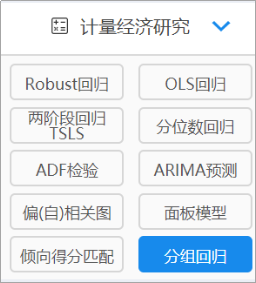分组回归

分别将薪水、福利、同事关系三项放入X框；学历放入分组项，工作忠诚度放入Y框。点击开始分析。分组回归

三、结果分析
（1）分组回归模型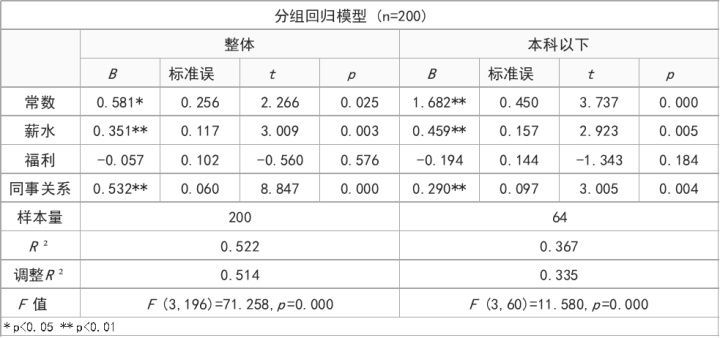表1：分组回归模型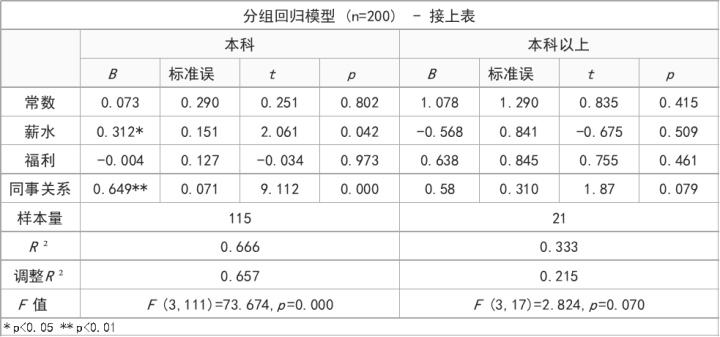表1：分组回归模型

上表展示的是，分组回归结果以及不同模型的比较情况。图中共有四个模型分别是：整体回归模型，以及学历分别为本科以下，本科和本科以上时3个组别单独得到的回归结果。
从每个模型的样本量中，也可以看出第一个模型为整体模型（样本量200），另外3个模型样本量总和为200。相当于分别筛选出对应学历的样本进行线性回归分析，其中本科以下样本为64人，本科为115人，本科以上21人。由于分组回归会分散每个回归的有效样本量，建议每个组别样本量较多时使用。
如何判断组间系数的差异（即是否有调节作用）：
分组回归可分为两步，第一步先针对整体模型进行分析，第二步针对不同组别时的回归模型结果进行分析。分析时可能出现以下情况：
①情况1：当出现整体模型中X对于Y没有影响。即说明X对Y没有影响（不显著，p 值大于0.05），那么第二步基本无意义。
②情况2：当整体模型通过F检验，X对Y有影响。第二步出现一部分组别显著一部分不显著，那么可以直接以某组别时X对Y有影响，某组别时X对Y无影响，作为研究结论（即有调节作用）。
③情况3：当整体模型通过F检验，X对Y有影响。第二步检验中每个组别下都有显著的影响，需要进一步针对回归系数的差异进行检验，如果回归系数具有显著性差异，则有调节效应，反着无调节效应。
根据上表结果，对整体回归模型进行分析：模型通过F检验，说明模型具有统计学意义，薪水和同事关系对工作忠诚度有正向影响关系。
对各分组回归模型进行分析：
当样本学历为本科以下时，薪水和同事关系会正向影响工作忠诚度；
当样本学历为本科水平时，薪水和同事关系会正向影响工作忠诚度；
当学历本科以上水平时，薪水、福利、同事关系均不会对工作忠诚度产生影响，即说明本科以上水平的样本，对于薪水、福利、同事关系的态度，并不会对他们的工作忠诚度产生影响。
（2）回归系数差异检验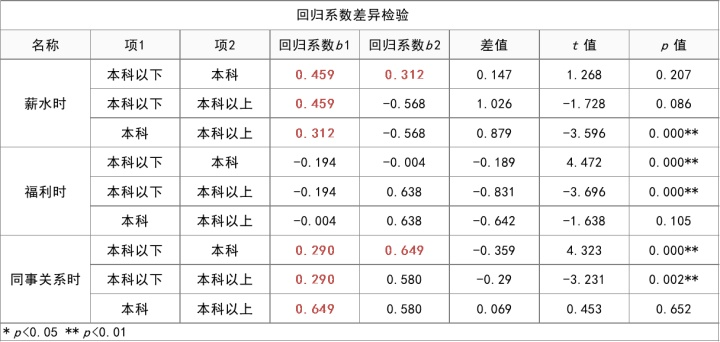表2：回归系数差异检验

回归系数差异检验结果是对上表结果的补充。如果在上一步回归模型结果中，显示在每个组别下X对于Y均有影响（显著，p 值小于0.05），那么可以通过检验对比回归系数，查看影响幅度是否存在的差异。
如果回归系数的差异显著，则在不同学历情况下，X对Y的影响幅度有显著性差异。即说明学历在X对Y的影响中起到了调节作用。
上表格展示‘薪水’,‘福利’和‘同事关系’分别对于工作忠诚度的影响，以及区分不同学历情况下时的回归系数差异情况。表格中红色部分表示在表1回归模型中有显著影响的结果。
薪水对于工作忠诚度的影响，本科以下和本科时，薪水对忠诚度都有显著的正向影响，此时可对比此2个回归系数（0.459和0.312）的差异幅度（t ＝1.268，p =0.207>0.05）,说明本科以下，本科时，薪水对于忠诚都有正向影响，影响幅度并没有明显的差异。
同事关系对于工作忠诚度的影响，本科以下或本科时，同事关系对忠诚度都有着正向影响（回归系数分别是0.290和0.649），而且影响幅度有着显著的差异（t ＝4.323，p =0.000< 0.01）,说明同事关系对忠诚度有着正向影响，而且本科学历（相对本科以下时）的影响幅度明显更大。
总结：综上所述，学历在薪水、同事关系对工作忠诚度的影响关系中起到了调节作用。
薪水对于工作忠诚度的影响，在学历为本科以上或本科时，薪水对忠诚度都有显著的正向影响，学历为本科时以上并没有影响。
同事关系对于工作忠诚度的影响，在学历为本科以下或本科时，同事关系都会对忠诚度有着正向影响，且本科学历时同事关系的影响幅度明显更大；但是本科以上学历样本时，同事关系对忠诚度不会产生影响。
四、其他说明
分组回归可用于研究调节作用，同时SPSSAU也提供分层回归分析（进阶方法->分层回归）或直接使用SPSSAU调节作用（问卷研究->调节作用）。
以上就是本次分享的内容，登录SPSSAU官网了解更多。

SPSSAU：如何分析调节作用？​zhuanlan.zhihu.comSPSSAU | 在线SPSS分析软件​www.spssau.com?100001000展开全文•   回归分析就是具有相关关系的两个或两个以上变量之间数量变化的一般关系进行测定，确定因变量和自变量之间数量变动的数学表达式，以便因变量进行估计或预测的统计分析方法。回归分析的数学描述如下：   ...

本篇博客的重点不在于回归模型的建立，而在于模型建立后的显著性检验。

1.回归分析
1.1 定义
回归分析就是对具有相关关系的两个或两个以上变量之间数量变化的一般关系进行测定，确定因变量和自变量之间数量变动的数学表达式，以便对因变量进行估计或预测的统计分析方法。回归分析的数学描述如下：   假设变量

x

1

,

x

2

,

x

3

,

…

,

x

k

x_{1},x_{2},x_{3},\dots,x_{k}

与随机变量

y

y

之间存在较显著的相关关系，则就有以下的回归模型：

y

=

f

(

x

1

,

x

2

,

x

3

,

…

,

x

k

)

+

ϵ

y=f(x_{1},x_{2},x_{3},\dots,x_{k})+\epsilon

其中

ϵ

\epsilon

为随机变量。而常用的回归模型是以下的线性形式：

y

=

β

0

+

β

1

x

1

+

β

2

x

2

+

⋯

+

β

k

x

k

+

ϵ

y=\beta_{0}+\beta_{1}x_{1}+\beta_{2}x_{2}+\cdots+\beta_{k}x_{k}+\epsilon

1.2 步骤
(1)根据研究目的和现象之间的内在联系，确定自变量和因变量。   做回归分析时，应该首先从理论出发进行定性分析，根据现象的内在联系确定变量之间的因果关系，从而确定哪个为自变量，哪个为因变量。 (2)确定回归分析模型的类型及数学表达式。   根据现象之间的内在影响机制或通过对具体变量数据描点分析，找出最适合的回归分析模型，再通过计算求出模型的待估参数，得到回归方程。常用的估计方法有普通最小平方法、岭回归、偏最小平方法回归、约束最小平方法回归，主成分回归。 (3)对回归分析模型进行评价和诊断。   得到具体的回归方程以后，要对其进行统计检验，来对回归方程的代表性及拟合程度进行拟合。
2.回归方程的统计检验
对于所拟合的回归模型，需要检验其合理性。检验的内容包括：模型的参数取值含义是否符合经济意义，模型的参数在统计意义上是否显著，模型整体的拟合效果是否理想，模型的假设条件是否满足。
2.1 模型整体拟合效果的显著性检验
记实际观察值为

y

i

y_{i}

，回归方程拟合的理论值(预测值)为

y

c

i

y_{c_{i}}

,观察值的平均值为

y

‾

\overline{y}

,则在最小平方法之下

y

c

‾

\overline{y_{c}}

与

y

‾

\overline{y}

是相等的。根据方差分析原理，则有

∑

(

y

−

y

‾

)

2

=

∑

(

y

c

−

y

‾

)

2

+

∑

(

y

−

y

c

)

2

\sum(y-\overline{y})^{2}=\sum(y_{c}-\overline{y})^{2}+\sum(y-y_{c})^{2}

或

S

S

T

=

S

S

R

+

S

S

E

SST=SSR+SSE

在误差项服从正太分布的情况下，应该有

S

S

R

∼

χ

2

(

k

)

,

S

S

E

∼

χ

2

(

n

−

k

−

1

)

SSR\sim\chi^{2}(k),SSE\sim\chi^{2}(n-k-1)

所以有

F

F

统计量

F

=

S

S

R

/

k

S

S

E

/

(

n

−

k

−

1

)

∼

F

(

1

,

n

−

k

−

1

)

F=\frac{SSR/k}{SSE/(n-k-1)}\sim F(1,n-k-1)

显然，回归方程拟合效果越好，表明方程解释部分所占比重越大，

S

S

R

SSR

和

S

S

E

SSE

相比的值也越大，

F

F

统计量也越大。因此，方程整体显著性检验的假设为：

H

0

:

β

0

=

β

1

=

β

2

=

⋯

=

β

k

=

0

H_{0}:\beta_{0}=\beta_{1}=\beta_{2}=\dots=\beta_{k}=0

H

1

:

β

0

≠

0

或

β

1

≠

0

或

…

或

β

k

≠

0

H_{1}:\beta_{0}\neq0或\beta_{1}\neq0或\dots或\beta_{k}\neq0

再给定显著性水平

α

\alpha

下，若

F

>

F

α

(

1

,

n

−

2

)

F>F_{\alpha}(1,n-2)

则拒绝原假设，认为回归方程整体是显著的。
2.2 模型参数显著性检验
模型参数显著性检验主要是判断每一个自变量对于回归模型是否必要。对于回归方程的单个参数进行

t

t

检验，对于回归方程中的每个参数

β

i

\beta_{i}

，其假设如下：

H

0

i

:

β

i

=

0

,

i

=

0

,

1

,

…

,

k

H_{0i}:\beta_{i}=0,i=0,1,\dots,k

H

1

i

:

β

i

≠

0

,

i

=

0

,

1

,

…

,

k

H_{1i}:\beta_{i}\neq0,i=0,1,\dots,k

其

t

t

检验统计量为：

t

=

β

i

σ

^

y

x

c

i

i

∼

t

(

n

−

k

−

1

)

t=\frac{\beta_{i}}{ \widehat \sigma_{yx}\sqrt{c_{ii}}}\sim t(n-k-1)

其中

c

i

i

c_{ii}

为矩阵

(

X

T

X

)

−

1

(X^{T}X)^{-1}

对角线上第

i

i

个元素，

σ

^

y

x

\widehat \sigma_{yx}

的计算公式如下：

σ

^

y

x

=

∑

(

y

−

y

c

)

2

n

−

k

−

1

\widehat \sigma_{yx}=\sqrt{\frac{\sum(y-y_{c})^{2}}{n-k-1}}

python中的statsmodel包中已经集成了完整的回归方程显著性检验。具体示例如下：
import pandas as pd
from scipy import stats
import statsmodels.api as sm
import numpy as np

X=pd.DataFrame(boston.data,columns=boston.feature_names)
y=pd.DataFrame(boston.target,columns=['target'])

model=sm.OLS(y,X)
results=model.fit()
y_pred=pd.DataFrame(model.predict(results.params,X),
columns=['pred'])
print(results.summary())

"""
自写代码计算过程
SSR=np.dot(y_pred['pred']-y['target'].mean(),y_pred['pred']-y['target'].mean())
SSE=np.dot(y_pred['pred']-y['target'],y_pred['pred']-y['target'])

(1)回归方程显著性检验
f_val=(SSR/13)/(SSE/492)
f_pval=stats.f.sf(f_val,13,492)

(2)单个参数的显著性检验
ttest_result=pd.DataFrame(None,index=X.columns,columns=['tval','pval'])
error=np.dot(y['target']-y_pred['pred'],y['target']-y_pred['pred'])
S = np.array(np.linalg.inv(np.dot(np.mat(X).T,X)))
for i,col in enumerate(X.columns):
tval=results.params[col]/np.sqrt((error/492)*S[i][i])
ttest_result.loc[col,'tval']=tval
pval=stats.t.sf(np.abs(tval),df=492)*2
ttest_result.loc[col,'pval']=pval
"""

其结果如下：从上述试验结果中可以发现，变量INDUS和AGE对回归方程是不显著的，可以从回归方程中剔除这两个变量。
参考资料
https://www.cnblogs.com/tangxianwei/p/8323495.htmlhttps://blog.csdn.net/u011517132/article/details/105286113
展开全文• 多元线性回归及显著性检验Matlab程序完美版 一说明 1本程序是硕士教材数理统计杨虎刘琼钟波 编著 例4.4.1P133Matlab编程解答程序教材上例题只做了回归方程显著性分析和一次回归系数显著性分析剔除x1后没有再检验x2和...
• 现在用 Python 写线性回归的博客都快烂大街了，为什么还要用 SPSS 做线性回归呢？这就来说说 SPSS 存在的原因吧。 SPSS 是一个很强大的软件，不用编程，不用调参，点巴两下就出结果了，而且出来的大多是你想要的。...数据建模 Python
• 今天小编就为大家分享一篇python 线性回归分析模型检验标准--拟合优度详解，具有很好的参考价值，希望大家有所帮助。一起跟随小编过来看看吧
• 线性与非线性回归模型检验方法研究，李武选，，在进行回归分析建模时，模型的准确性是极其重要的，不恰当的模型，会歪曲所研究问题的事实真相，误导人们问题的正确理解。在取
• 本资源适用于做二元线性回归、残差分析的人有所帮助。如：做ndvi与温度、降雨的线性回归问题做残差分析。
• 回归分析的结果展示 一致的有回归结果对比图 RMSE 回归图 误差直方图matlab
• ## stata 线性回归分析基本操作

万次阅读 多人点赞 2019-09-18 09:55:53
一、线性回归基本命令 regress y x1 x2 （红色... 表上半部分为方差分析表，包括回归平方和，残差平方和，均方，F检验等。上半部分右侧给出拟合优度R2和调整的R2。root MSE 表示方程的标准误差。 表下半部分为...
• ## SPSS篇—回归分析

万次阅读 多人点赞 2019-08-20 09:29:06
今天给大家分享一下如何用SPSS Statistics来进行回归分析，我们通过一个实例来具体了解一下整个分析的过程以及结果的解读。 上图中就是本次需要进行回归分析的数据，之前有跟大家说过，SPSS Statistics的界面跟...SPSS数据分析 数据分析 SPSS分析
• 为了能够比较直观地了解到三种回归方法的区别，本文基于李子奈、潘文卿的《计量经济学》（第四版）第四章的同一案列进行三种方法的结果比较，具体推导过程可参见文后提供的参考链接。 首先导入需要用到的库 ...
• ## spss进行多元线性回归并分析表格

万次阅读 多人点赞 2019-04-01 00:50:26
1.如何使用spss进行多元线性回归。 2.分析生成结果，并判断回归是否可行。 一、使用spss进行多元线性回归： 1.输入数据 2. 3. 4. 5. 6. 二、表格结果分析： R方是检验回归是否成功的重要要素...
• 终于找到一篇全面而又简洁的讲多元线性回归模型检验方法的文章，涵盖了 主要的统计检验——F检验、t检验、DW检验
• ## Eviews回归结果解读

万次阅读 2021-03-05 14:43:54
最近涨了很多粉，看来大家eviews的操作都很有兴趣，鉴于关注我的大多数是我的小白学生客户，所以我整理了一些基础知识帮大家快速入门，方便大家读懂模型结果。 简单的多元线性回归模型大家是使用最多的，但是很多...
• 使用EViews软件进行OLS估计参数，建立线性回归模型，同时得到模型的拟合图和残差图。点击proc->Make Model，弹出Model窗口。得到回归方程。 演示数据为股票数据:上证指数与微博情感分析处理后的大V指数数据之间的...eviews 股票数据分析 OLS
• 然后，采用滑动窗口机制截取的视频帧提取HOG特征并利用过训练好的逻辑回归模型进行检测，并结合非极大值抑制技术去除了多余的检测窗口；通过实验，在车辆图像的测试集上该模型的识别正确率达到了96.23%，在视频帧...
• 2.用Python底层编写进行计量经济分析（二）：多重共线性（原因、结果检验：条件数/方差膨胀因子、补救：岭回归） 模型符合线性模式 XXX满秩（无多重共线） 零均值价值：E(εi∣Xi)=0E(ε_i|X_i)=0E(εi​∣Xi​)=...数据分析 统计学 统计模型
• 文章目录 十一、回归方程与回归系数的显著性检验 1.平方和分解 2.回归方程的假设检验 3.中心化的等价形式 4.回归系数的假设检验 5.回归方程的预报精度 回顾总结 十一、回归方程与回归系数的显著性检验 回归方程的...多元统计分析
• ## STATA regress回归结果分析

万次阅读 多人点赞 2020-07-30 22:37:11
对于STATA回归结果以前一直不清不楚，每次都需要baidu一波，因此今天将结果相关分析记录下： 如上图 上面左侧的表是用来计算下面数据的，分析过程中基本不会用到 右侧从上往下 1.Number of obs 是样本容量 2.F是...数据分析 1024程序员节
• 本文主要介绍：Excel数据分析工具库—回归线性回归和非线性回归简单线性回归和多重线性回归逻辑斯蒂回归一、什么是回归分析(Regression)1、定义确定两种或两种以上变量间相关关系的一种统计分析方法。通过数据间...相关系数excel
• ## Python 多元回归实现与检验

万次阅读 多人点赞 2018-06-06 11:03:42
decision_function(X) 和predict(X)都是利用预估器训练数据X进行预测，其中decision_function(X)包含了输入数据的类型检查，以及当前对象是否存在coef_属性的检查，是一种“安全的”方法，而predict是decision...
• 在他的帮助文档中，很好的总结了如何比较拟合结果的好坏 以下为具体网址：...
• 实现分布滞后分位数回归，包括方差标准差，t检验各系数是否显著
• 回归分析是数据分析中最基础也是最重要的分析工具，绝大多数的数据分析问题，都可以使用回归的思想来解决。回归分析的任务就是， 通过研究自变量X和因变量Y的相关关系，尝试去解释Y的形成机制，进而达到通过X去预测Y...多重共线性
• 系列前面的文章： 1.用Python底层编写进行计量经济分析...3用Python底层编写进行计量经济分析（三）：异方差（原因、结果检验：White检验、补救：广义线性回归） 多元线性回归的基本假定： 模型符合线性模式 XX...数据分析 统计学 统计模型
• 1、系数显著性检验，t检验 2、方程显著性检验，F检验 2.1、模型线性性检验（和2中适用于不同情况）：LM（拉格朗日）检验、Wald检验、LR（似然比）检验 3、拟合有度R2，adjust-R2 4、多重共线性检验，VIF值 5、......